## Design Multirate Filters

Note

If you are using R2016a or an earlier release, replace each call to the object with the equivalent step syntax. For example, `obj(x)` becomes `step(obj,x)`.

Multirate filters are filters in which different parts of the filter operate at different rates. Such filters are commonly used when the input and output sample rates differ, such as during decimation, interpolation, or a combination of both. However, multirate filters are often used in designs where the input sample rate and output sample rate are the same. In such filters, there is an internal decimation and interpolation occurring in a series of filters. Such filters can achieve both greatly reduced filter lengths and computational rates as compared to standard single-rate filter designs.

The most basic multirate filters are interpolators, decimators, and rate converters. These filters are building components of more advanced filter technologies such as filter banks and Quadrature Mirror Filter (QMF). You can design these filters in MATLAB® and Simulink® using the `designMultirateFIR` function.

The function uses the FIR Nyquist filter design algorithm to compute the filter coefficients. To implement these filters in MATLAB, use these coefficients as inputs to the `dsp.FIRDecimator`, `dsp.FIRInterpolator`, and `dsp.FIRRateConverter` System objects. In Simulink, compute these coefficients using `designMultirateFIR` in the default Auto mode of the FIR Decimation, FIR Interpolation, and FIR Rate Conversion blocks.

The inputs to the `designMultirateFIR` function are the interpolation factor and the decimation factor. Optionally, you can provide the half-polyphase length and stopband attenuation. The interpolation factor of the decimator is set to 1. Similarly, the decimation factor of the interpolator is set to 1.

These examples show how to implement an FIR decimator in MATLAB and Simulink. The same workflow can apply to an FIR interpolator and FIR rate converter as well.

### Implement an FIR Decimator in MATLAB

To implement an FIR Decimator, you must first design it by using the `designMultirateFIR` function. Specify the decimation factor of interest (usually greater than 1) and an interpolation factor equal to 1. You can use the default half-polyphase length of 12 and the default stopband attenuation of 80 dB. Alternatively, you can also specify the half-polyphase length and stopband attenuation values.

Design an FIR decimator with the decimation factor set to 3 and the half-polyphase length set to 14. Use the default stopband attenuation of 80 dB.

```b = designMultirateFIR(1,3,14); ```

Provide the coefficients vector, `b`, as an input to the `dsp.FIRDecimator` System object?.

```FIRDecim = dsp.FIRDecimator(3,b); fvtool(FIRDecim); ```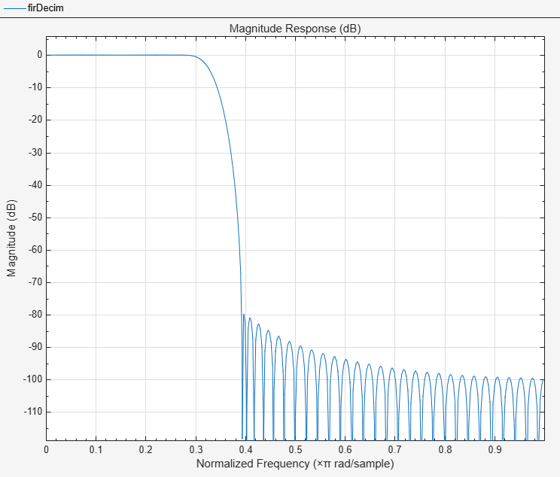By default, the `fvtool` shows the magnitude response. Navigate through the `fvtool` toolbar to see the phase response, impulse response, group delay, and other filter analysis information.

Filter a noisy sine wave input using the `FIRDecim` object. The sine wave has frequencies at 1000 Hz and 3000 Hz 3000 Hz. The noise is a white Gaussian noise with mean zero and standard deviation 1e-5. The decimated output will have one-third the sample rate as input. Initialize two `dsp.SpectrumAnalyzer` System objects, one for the input and the other for the output.

```f1 = 1000; f2 = 3000; Fs = 8000; source = dsp.SineWave('Frequency',[f1,f2],'SampleRate',Fs,... 'SamplesPerFrame',1026); specanainput = dsp.SpectrumAnalyzer('SampleRate',Fs,... 'PlotAsTwoSidedSpectrum',false,... 'ShowLegend',true,'YLimits',[-120 30],... 'Title','Noisy Input signal',... 'ChannelNames', {'Noisy Input'}); specanaoutput = dsp.SpectrumAnalyzer('SampleRate',Fs/3,... 'PlotAsTwoSidedSpectrum',false,... 'ShowLegend',true,'YLimits',[-120 30],... 'Title','Filtered output',... 'ChannelNames', {'Filtered output'}); ```

Stream in the input and filter the signal in a processing loop.

```for Iter = 1:100 input = sum(source(),2); noisyInput = input + (10^-5)*randn(1026,1); output = FIRDecim(noisyInput); specanainput(noisyInput) specanaoutput(output) end ```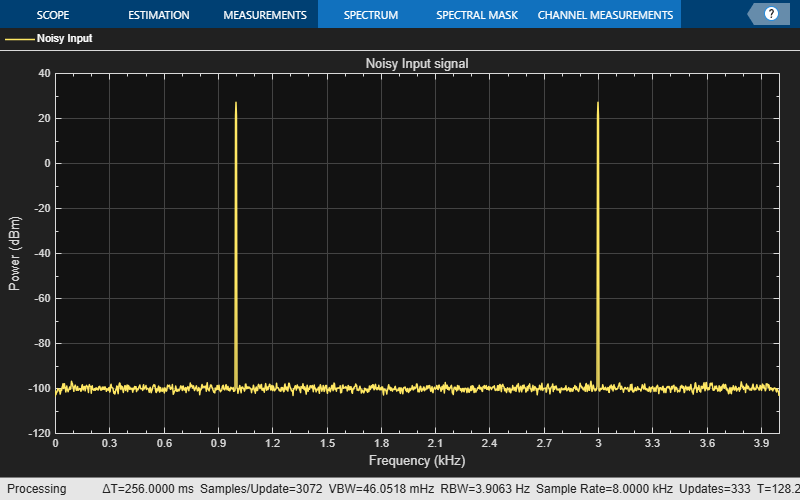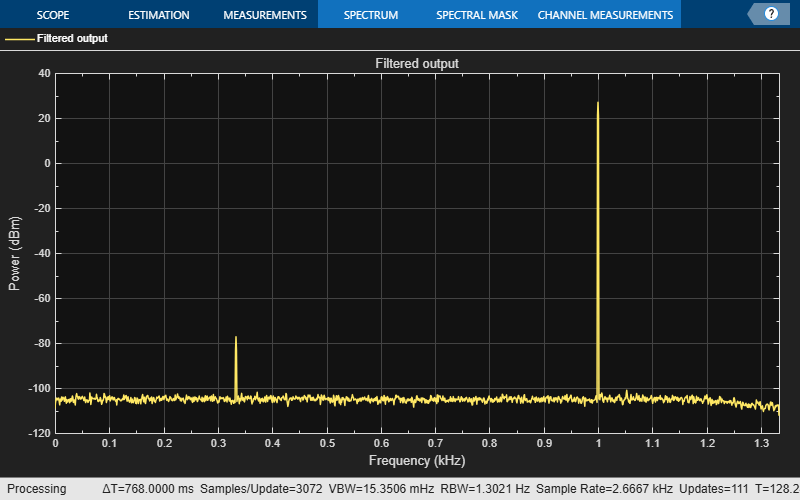The input has two peaks: one at 1000 Hz and the other at 3000 Hz. The filter has a lowpass response with a passband frequency of 0.3*pi rad/sample. With a sampling frequency of 8000 Hz, that is a passband frequency of 1200 Hz. The tone at 1000 Hz is unattenuated, because it falls in the passband of the filter. The tone at 3000 Hz is filtered out.

Similarly, you can design an FIR interpolator and FIR rate Converter by providing appropriate inputs to the `designMultirateFIR` function. To implement the filters, pass the designed coefficients to the `dsp.FIRInterpolator` and `dsp.FIRRateConverter` objects.

### Implement an FIR Decimator in Simulink

You can design and implement the FIR multirate filters in Simulink using the FIR Decimation, FIR Interpolation, and FIR Rate Conversion blocks. When you set Coefficient source to Dialog parameters, you can provide `designMultirateFIR(1,2)` as a parameter to specify the filter coefficients. To design the Decimator using the `designMultirateFIR` function, you must specify the decimation factor of interest (usually greater than 1) and an interpolation factor equal to 1. You can use the default half-polyphase length of 12 and the default stopband attenuation of 80 dB. Alternatively, you can also specify the half-polyphase length and stopband attenuation values.The block chooses the coefficients computed using the `designMultirateFIR` function.

Similarly, you can design an FIR interpolator and FIR rate converter by providing appropriate inputs to the `designMultirateFIR` function.

When you set Coefficient source to Auto, the block computes the coefficients using the `designMultirateFIR` function. The function uses the decimation factor specified in the block dialog box.You can design an FIR Interpolator and an FIR Rate Converter using a similar approach in the corresponding blocks.

### Sample Rate Conversion

Sample rate conversion is a process of converting the sample rate of a signal from one sampling rate to another sampling rate. Multistage filters minimize the amount of computation involved in a sample rate conversion. To perform an efficient multistage rate conversion, the `dsp.SampleRateConverter` object:

1. accepts input sample rate and output sample rate as inputs.

2. partitions the design problem into optimal stages.

3. designs all the filters required by the various stages.

4. implements the design.

The design makes sure that aliasing does not occur in the intermediate steps.

In this example, change the sample rate of a noisy sine wave signal from an input rate of 192 kHz to an output rate of 44.1 kHz. Initialize a sample rate converter object.

```SRC = dsp.SampleRateConverter; ```

Display the filter information.

```info(SRC) ```
```ans = 'Overall Interpolation Factor : 147 Overall Decimation Factor : 640 Number of Filters : 3 Multiplications per Input Sample: 27.667188 Number of Coefficients : 8631 Filters: Filter 1: dsp.FIRDecimator - Decimation Factor : 2 Filter 2: dsp.FIRDecimator - Decimation Factor : 2 Filter 3: dsp.FIRRateConverter - Interpolation Factor: 147 - Decimation Factor : 160 ' ```

`SRC` is a three-stage filter: two FIR decimators followed by an FIR rate converter.

Initialize the sine wave source. The sine wave has two tones: one at 2000 Hz and the other at 5000 Hz.

```source = dsp.SineWave ('Frequency',[2000 5000],'SampleRate',192000,... 'SamplesPerFrame',1280); ```

Initialize the spectrum analyzer to see the input and output signals.

```Fsin = SRC.InputSampleRate; Fsout = SRC.OutputSampleRate; specanainput = dsp.SpectrumAnalyzer('SampleRate',Fsin,... 'PlotAsTwoSidedSpectrum',false,... 'ShowLegend',true,'YLimits',[-120 30],... 'Title','Input signal',... 'ChannelNames', {'Input'}); specanaoutput = dsp.SpectrumAnalyzer('SampleRate',Fsout,... 'PlotAsTwoSidedSpectrum',false,... 'ShowLegend',true,'YLimits',[-120 30],... 'Title','Rate Converted output',... 'ChannelNames', {'Rate Converted output'}); ```

Stream in the input signal and convert the signal's sample rate.

```for Iter = 1 : 5000 input = sum(source(),2); noisyinput = input + (10^-5)*randn(1280,1); output = SRC(noisyinput); specanainput(noisyinput); specanaoutput(output); end ```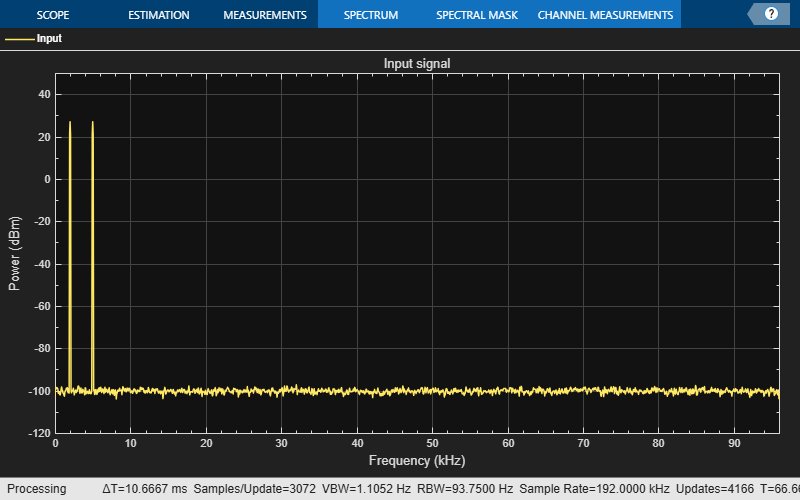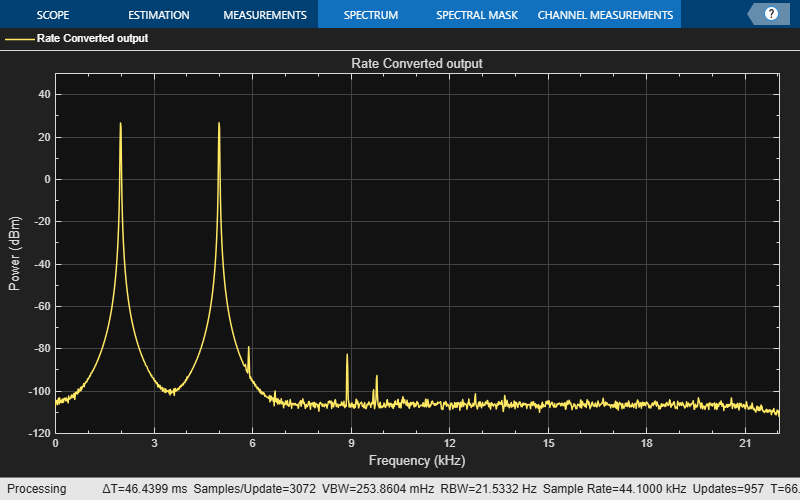The spectrum shown is one-sided in the range [0 Fs/2]. For the spectrum analyzer showing the input, Fs/2 is 192000/2. For the spectrum analyzer showing the output, Fs/2 is 44100/2. Hence, the sample rate of the signal changed from 192 kHz to 44.1 kHz.

 Harris, Fredric J. Multirate Signal Processing for Communication Systems. Prentice Hall PTR, 2004.

Watch now